# What power

What power should the radiator have in a room 5x4 meters with a height of 3 meters?

Hint:
Calculate 35 watts per meter ^ 3 in case you need 22 degrees in the room but you need about 24 degrees in the bathroom. It's an old heating cheat, but it will definitely help you.

P =  2100 W

### Step-by-step explanation: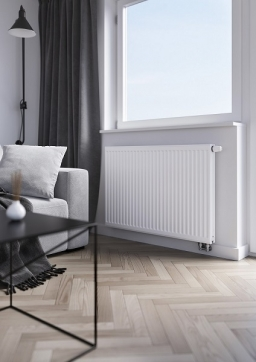Did you find an error or inaccuracy? Feel free to write us. Thank you!Tips to related online calculators
Do you know the volume and unit volume, and want to convert volume units?

## Related math problems and questions:

• Concrete columnThe concrete column of the highway bridge has the shape of a block with dimensions of 1m x 0.8m x 25m. It should be lifted by crane to a height of 20m. What is the power of his engine if the lifting takes 2 minutes?
• Latent heat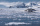How much heat is needed to take from 100 g of water at 20°C to cool to ice at -18°C? Mass heat capacity c (ice) = 21kJ/kg / °C; c (water) = 4.19 KJ/kg / °C and the mass group heat of solidification of water is l = 334kJ/kg
• ThereThere is 5 l of water with a temperature of 20°C in the bucket. We add 3 liters of hot water. The resulting temperature will be 30°C. What was the temperature of the hot water?
• Air thermal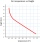Imagine that a unit of air rises at 3000 meters high, if temperature decreases 6 degrees celcius for every 1000 meter, what will be its temperature at 1400 meters, 2000 meters, 2500 meters and when it reaches the 3000 meter elevation. Starting temperature
• FreezerThe freezer has the shape of a cuboid with internal dimensions of 12 cm, 10 cm, 30 cm. A layer of ice of 23 mm thick was formed on the inner walls (and on the opening) of the freezer. How many liters of water will drain if we dispose the freezer?
• Motor physics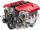A car is traveling at 98 km/h on the highway. The pulling power of the motor is 6 kN. Find the engine power in kW.
• Ice and water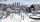We want to cover rectangular rink with dimensions of 55 m and 25 m with 4cm thick layer of ice. How many liters of water we need if after freezing water increases its volume by 10%?
• Cuboid aquariumCuboid 25 times 30 cm. How long is third side if cuboid contains 30 liters of water?
• Overtaking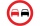On the direct road, the passenger car overtakes the slower bus by starting to overtake 20 meters from the bus and after passing it ahead of it again 20 meters away. The car overtakes at a steady speed of 72 km/h, the bus goes at a steady speed of 54 km/h.
• WeatherWeather. At 5:00 am, the temperature was 28°C, and at noontime, it rose by 9°C. By 4:00 pm, it dropped 3°C, and by nighttime, it was 4°C less. What is the room temperature this time?
• Water temperaturesTo 25 liters of water at 50 °C we add 15 liters of water at a different temperature. How many °C should the water be colder than 50 °C so that 40 liters of the water obtained has a temperature 42.5 °C?
• Water mixingWe have 520 ml of hot water and 640 ml of water at 48°C. What is the temperature of approximately hot water when the resulting mixture has a temperature of 65°C?
• A residentialA residential colony has a population of 5400, and 60 liters of water is required per person per day. The effective utilization of rainwater constructed a water reservoir measuring 48m × 27m × 25m to collect the rainwater. For how many days, the water of
• SeawaterSeawater has a density of 1025 kg/m3, ice 920 kg/m3. 8 liters of seawater froze and created a cube. Calculate the size of the cube edge.
• Electric workCalculate the work done by the electric forces passing the current of 0.2 A through the bulb in 10 minutes if the bulb is connected to a 230 V power supply.
• The bathThe bath has water with a volume of 220 l and a temperature of 65°C. Determine the mass m2 of water at 15°C to be poured so that the resulting temperature of the water in the bath is 40°C. We neglect the heat exchange between water and the environment. T
• Workers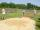Workers digging a jump pit in the schoolyard. The pit has a cuboid shape with a length 12 m, a width of 20 dm, and a depth of 36 cm. They excavate 0.4 cubic meters of soil an hour. How much time (hours and minutes) is need to excavate this pit?